• ## Eigenvalue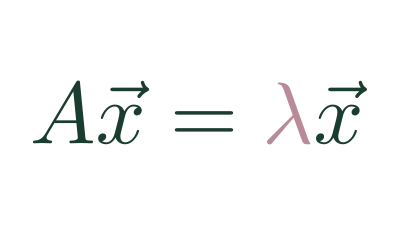A value which describes how much a transformation scales an Eigenvector

• ## Eigenvector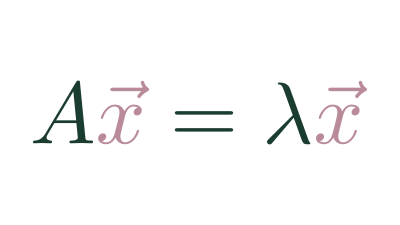A set of vectors whose span doesn't change after a transformation.

• ## Changing Basis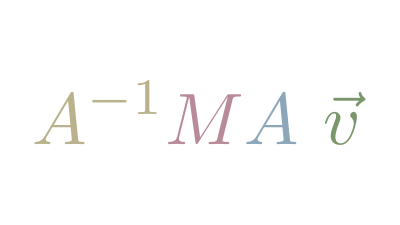Since any vectors can be Basis Vectors, it's useful to understand how to translate vectors between bases

• ## Cross Product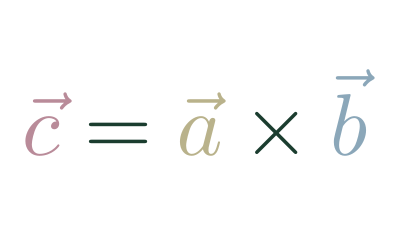An operation between two 3d vectors that returns a vector.

• ## Matrix Determinate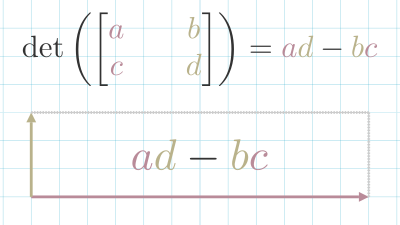A measure of how a matrix scales space.

• ## Matrix Transformation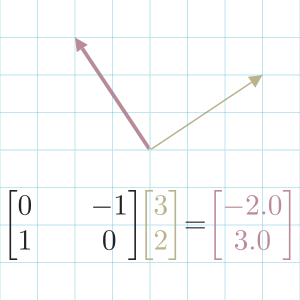A matrix as a transformation of a space.

• ## Basis Vectors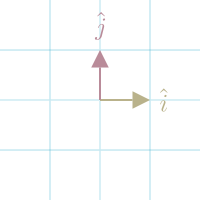The set of vectors that defines space.

• ## Matrix Inverse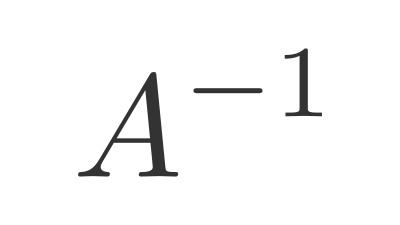A matrix that reverses a transformation.

• ## Identity Matrix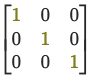When you multiply a matrix $(A)$ by the Identity Matrix $(I)$, you get the original matrix back.

$A …$

• ## Matrix Multiplication

Matrix multiplication is a mathematical operation between 2 matrices that returns a matrix.

For each row in the first matrix, take the Dot Productf …